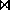Next: Use of an Index Up: Join Strategies Previous: Block-Oriented Iteration

Merge-Join

1. Suppose neither relation fits in main memory, and both are stored in sorted order on the join attributes. (E.g. both deposit and customer sorted by cname.)
2. We can then perform a merge-join, computed like this:
• Associate one pointer with each relation.
• Initially these pointers point to the first record in each relation.
• As algorithm proceeds, pointers move through the relation.
• A group of tuples in one relation with the same value on the join attributes is read.
• Then the corresponding tuples (if any) of the other relation are read.
• Since the relations are in sorted order, tuples with same value on the join attributes are in consecutive order. This allows us to read each tuple only once.
3. In the case where tuples of the relations are stored together physically in their sorted order, this algorithm allows us to compute the join by reading each block exactly once.
• For depositcustomer, this is a total of 510 block accesses.
• This is as good as the block-oriented method with the inner loop relation fitting into main memory.
• The disadvantage is that both relations must be sorted physically.
• It may be worthwhile to do this sort to allow a merge-join.

Osmar Zaiane
Sun Jul 26 17:45:14 PDT 1998